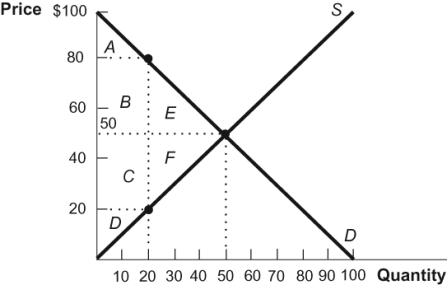# [Solved] Use the Following to Answer Questions

Question 80
Multiple Choice

## Use the following to answer questions: Figure: Price and Quantity 2-(Figure: Price and Quantity 2) At a cost of \$20 per unit in the diagram, the value of the unexploited gains from trade is represented by areas:

A) A + B + E.
B) A + B + C + D.
C) E + F.
D) B + C + E + F.

10+ million students use Quizplus to study and prepare for their homework, quizzes and exams through 20m+ questions in 300k quizzes.

Explore our library and get Economics Homework Help with various study sets and a huge amount of quizzes and questions

3.5K

Study sets

66.5K

Quizzes

5.9M

Questions

Upload material to get free accessInvite a friend and get free accessSubscribe and get an instant access